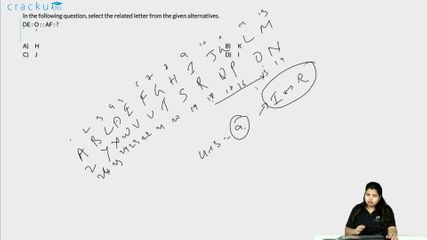Question 2

# In the following question, select the related letter from the given alternatives.DE : O : : AF : ?

Solution

Expression = DE : O : : AF : ?

Now, D = 4 , E = 5 and O = 15

The pattern followed is = $$(4+5)\times2-3=18-3=15$$

Similarly, A = 1 and F = 6

Thus, $$(1+6)\times2-3=14-3=11=K$$

=> Ans - (B)

### View Video Solution• Free SSC Study Material - 18000 Questions
• 230+ SSC previous papers with solutions PDF

##### Karobi Paik

3 years, 3 months ago

12:20::30:? How can i solved

##### Neeru Sehrawat

1 month, 2 weeks ago

12 : 20 :: 30 : ?
4*3=12, 4*5=20
6*3=18, 6*5=30